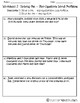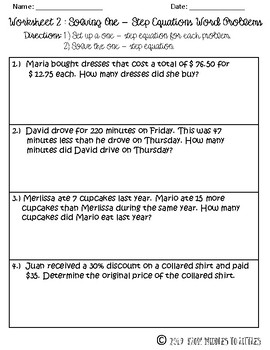# Solving One-Step Equations {Sweet Sheets}Subject
Resource Type
Format
PDF (823 KB|8 pages)
Standards
\$2.25

### Description

The following worksheet packet involves 3 pages of worksheets. Worksheet 1 (one page) involves computation of 10 one-step equations (including fractions, decimals, and negative numbers). Worksheet 2 (two pages) includes 8 word problems. For each real-life problem, students must set up the equation as well as solve for the variable.

Total Pages
8 pages
Included
Teaching Duration
N/A
Report this Resource to TpT
Reported resources will be reviewed by our team. Report this resource to let us know if this resource violates TpT’s content guidelines.

### Standards

to see state-specific standards (only available in the US).
Solve linear equations in one variable.
Use variables to represent quantities in a real-world or mathematical problem, and construct simple equations and inequalities to solve problems by reasoning about the quantities.
Solve multi-step real-life and mathematical problems posed with positive and negative rational numbers in any form (whole numbers, fractions, and decimals), using tools strategically. Apply properties of operations to calculate with numbers in any form; convert between forms as appropriate; and assess the reasonableness of answers using mental computation and estimation strategies. For example: If a woman making \$25 an hour gets a 10% raise, she will make an additional 1/10 of her salary an hour, or \$2.50, for a new salary of \$27.50. If you want to place a towel bar 9 3/4 inches long in the center of a door that is 27 1/2 inches wide, you will need to place the bar about 9 inches from each edge; this estimate can be used as a check on the exact computation.

### Q & A

Teachers Pay Teachers is an online marketplace where teachers buy and sell original educational materials.